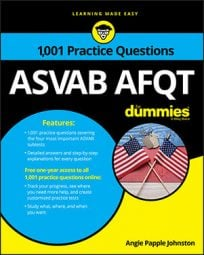##### ASVAB AFQTA great way to flex your algebra muscles is by tackling number sequence problems. On the Arithmetic Reasoning subtest on the ASVAB, these problems will usually require you to find consecutive numbers (odd or even) based on, for example, their sum.

## Practice questions

1. Two consecutive numbers have a sum of 109. What are the numbers?

A. 64 and 65 B. 54 and 55 C. 53 and 54 D. 52 and 56

2. Three consecutive odd numbers have a sum of 21. What are the three numbers?

A. 1, 3, and 5 B. 7, 9, and 11 C. 3, 5, and 7 D. 5, 7, and 9

1. The correct answer is Choice (B).

Let x represent the first number, and let x + 1 represent the second number. Then create your equation:

The first number is 54, and because the problem tells you that you need consecutive numbers (and because the second number is x + 1), you know the second number is 55.

Sometimes on the ASVAB, running through the answer choices is a good idea. You can immediately rule out Choice (D) because the numbers aren't consecutive, and you can rule out Choices (A) and (C) because they don't add up to 109.

2. The correct answer is Choice (D).

Let n represent the first odd number. Because the numbers are consecutive odd numbers, let n = 2 represent the second consecutive odd number, and then let n = 4 represent the third consecutive odd number. Create an equation and solve:

The first number is 5, which tells you that the next odd numbers in the sequence are 7 and 9.

On problems like this one, plugging in the likely answer choices may be faster. If they add up to the correct amount, you can choose quickly and move to the next problem. You have only 39 minutes to answer 16 questions on the Arithmetic Reasoning subtest of the CAT-ASVAB and 36 minutes to answer 30 questions on the paper-and-pencil version, so save time where you can.